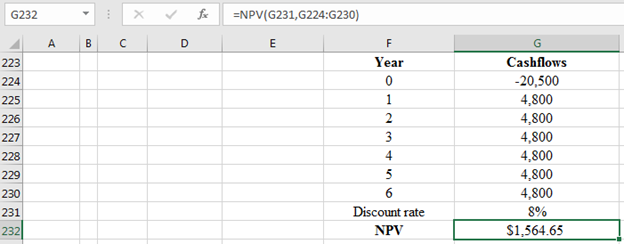# A new computer system will require an initial outlay of \$20,500, but it will increase the firm’s cash flows by \$4,800 a year for each of the next 6 years. a. Calculate the NPV and decide if the system is worth installing if the required rate of return is 8%. (Negative amount should be indicated by a minus sign. Do not round intermediate calculations. Round your answers to 2 decimal places.) b. Calculate the NPV and decide if the system is worth installing if the required rate of return is 13%. (Negative amount should be indicated by a minus sign. Do not round intermediate calculations. Round your answers to 2 decimal places.)

Question
12 views

A new computer system will require an initial outlay of \$20,500, but it will increase the firm’s cash flows by \$4,800 a year for each of the next 6 years.

a. Calculate the NPV and decide if the system is worth installing if the required rate of return is 8%. (Negative amount should be indicated by a minus sign. Do not round intermediate calculations. Round your answers to 2 decimal places.)

b. Calculate the NPV and decide if the system is worth installing if the required rate of return is 13%. (Negative amount should be indicated by a minus sign. Do not round intermediate calculations. Round your answers to 2 decimal places.)

check_circle

Step 1

The difference between the future cash inflows and future cash outflows will be regarded as net present value at a given required rate of return.

Given information:

Initial cash outlay is -\$20,500

Cash inflows for each of the next 6 years will be \$4,800

Discount rate is 8%

Step 2

Calculations:

(a)Step 3

The NPV is \$1,564.65

Interpretation:

At the given required rate of return, it is worth...

### Want to see the full answer?

See Solution

#### Want to see this answer and more?

Solutions are written by subject experts who are available 24/7. Questions are typically answered within 1 hour.*

See Solution
*Response times may vary by subject and question.
Tagged in